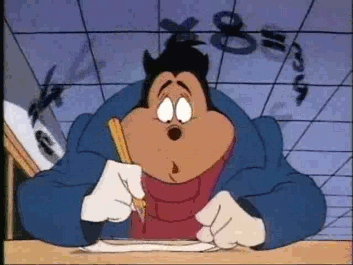## Maths - Spring 2019

IAt Holy Spirit we follow White Rose scheme which focuses on fluency, reasoning and problem solving.

In Autumn Term we will be focusing on Place value, Addition and Subtraction

All children in year 4 will be learning their times tables (x12) ready for the new test in the Summer term. Please help us with your child's maths learning by practicing their times tables with them regularly.

Place Value:

·         Recognise the place value of each digit in a four-digit number )thousands, tens and ones)

·         Recognise and be able to solve problems involving Roman Numerals

·         Count backwards through zero to include negative numbers

·         Order and compare numbers beyond 1000

·         Find 1000 more or less than a given number

·         Identify, represent and estimate numbers using different representations

·         Count in multiples of 6, 7, 9, 25 and 1000

·         Round any number to the nearest 10, 100, 1000

·         Read Roman numerals to 100 and know that over time, the numeral system changed to include the concept of zero and place value.

 ·         methods of column addition and subtraction when appropriate ·         Solve number and practical problems that involve all of the above with increasingly large positive numbers, number and place value. ·         Estimate and use inverse operations to check answers to a calculation ·         Solve addition and subtraction two-step problems in contexts, deciding which operations and methods to use and why Multiplication and Division ·         Recall multiplication and division facts for multiplication tables up to x12 ·         Use place value, known and derived facts to multiply and divide mentally. ·         Recognise and use factor pairs in mental calculations ·         Multiply two-digit and three-digit by a one digit number using formal method Fractions ·         Recognise and show using diagrams, families of common equivalent fractions ·         Count up and down in hundredths, recognise that hundredths arise when dividing an object by a hundred and dividing tenths by ten ·         Add and subtract fractions with the same denominator ·         Recognise and write decimal equivalents of any number of tenths or hundredths ·         Recognise and write decimal equivalents to quarters, halves and three quarters ·         Find the effect of dividing a one or two-digit number by 10 and 100, identifying the value of the digits in the answer as ones, tenths and hundredths. ·         Round decimals with one decimal place to the nearest whole number. ·         Compare numbers with the same number of decimal places up to two decimal places Continuous Objectives ·         Solve number and practical problems that involve all of the above and with increasingly large positive numbers number and place value. ·         Estimate and use inverse operations to check answers to a calculation. ·         Solve addition and subtraction two-step problems in contexts, deciding which operations and methods to use and why. ·         Solve problems, involving multiplying and adding, including using the distributive law to multiply two digit numbers by one digit. ·         Solve simple measure and money problems involving fractions and decimal problems to two decimal places. ·         Solve problems, involving converting from hours to minutes; minutes to seconds; years to months; weeks to days.

We will be learning how to solve times tables questions quickly using our New Fast Maths method of learning. During our FAST MATHS sessions we try to complete as many numbers on a multiplication grid as possible whilst beating our previous time. We love it!